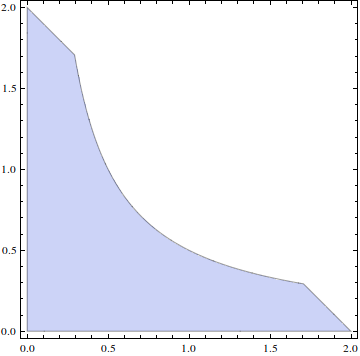# Strange region

Calculus Level 4

What is the area of the region defined by the following set of inequalities?

$\displaystyle \begin{array}{cc} (1)&0 < 2xy < 1 \\ (2)&0 < \frac{x+y}{2} < 1 \end{array}$

If the area is $A$, give $\left\lfloor100A\right\rfloor$.×